Definitions of Square Dance Calls and Concepts

Single Double Pass Thru, Diamond \$B\$+\$iA40w(B 1/4 In \$B\$7\$?(B formation, \$B\$^\$?\$OB>\$NE,@Z\$J(B formation \$B\$+\$i!%(B

Centers \$B\$,;O\$a\$N(B fraction \$B\$N(B Touch \$B\$r9T\$\$(B Spread \$B\$7\$FB>\$N?M\$OA0?J\$7(B, new Centers \$B\$Ofraction \$B\$N(B Touch \$B\$r9T\$&\$+(B anything \$B\$N%3!<%k\$r9T\$\$\$^\$9!%\$3\$N%3!<%k\$G\$O(B fraction \$B\$,>JN,\$5\$l\$?\$H\$-\$N%G%U%)%k%H\$O\$"\$j\$^\$;\$s!%(B
\$BLuJN,\$5\$l\$?\$H\$-\$K;H\$o\$l\$kCM\$r;X\$98@MU\$G(B,\$B!V(BSquare Thru \$B\$N%G%U%)%k%H\$O(B 4\$B!W\$N\$h\$&\$K;H\$\$\$^\$9!%(B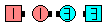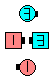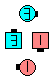Touch By 1/2 By 1/4\$B\$NA0(B Centers \$B\$,(B Touch 1/2 - Spread, \$BB>\$N?M\$O(B step forward \$B\$N8e(B new Centers Touch 1/4\$B\$N8e(B (\$B=*\$o\$j(B)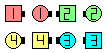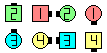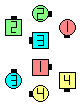Touch By 1/4 By Swing Thru\$B\$NA0(B Centers \$B\$,(B Touch 1/4 - Spread, \$BB>\$N?M\$O(B step forward \$B\$N8e(B new Centers Swing Thru\$B\$N8e(B (\$B=*\$o\$j(B)

\$BCmanything \$B\$N%3!<%k(B (2 \$B?M\$N%3!<%k\$G\$"\$kI,MW\$O\$"\$j\$^\$;\$s(B) \$B\$O8~\$+\$\$9g\$C\$?\$H\$3\$m\$+\$i9T\$\$\$^\$9!%(BDouble Pass Thru \$B\$+\$i(B, Touch By 1/4 By Recycle (facing Recycle) \$B\$r9M\$(\$F\$_\$F\$/\$@\$5\$\$!%(B

\$B1~MQ(B:

• Column of 6 Touch By (3 fractions \$B\$^\$?\$O(B anything \$B\$,M?\$(\$i\$l\$^\$9(B),
• Column of 8 Touch By (4 fractions \$B\$^\$?\$O(B anything \$B\$,M?\$(\$i\$l\$^\$9(B)\$B!%(B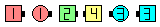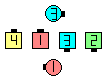Touch By 1/4 By 1/2 By 3/4\$B\$NA0(B \$B8e(B